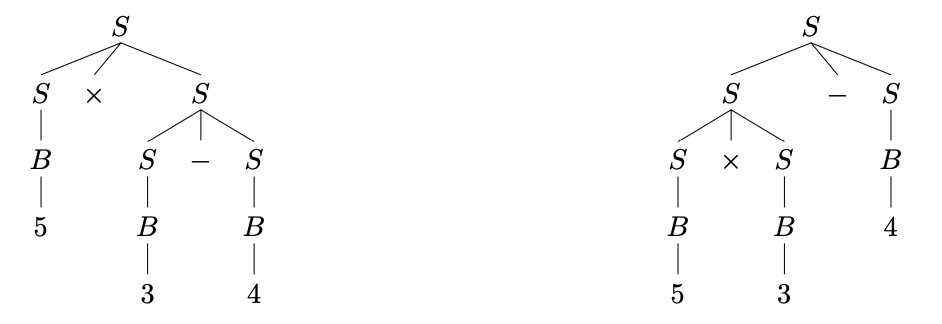# Ch3 计算理论 表述复杂语言

## Consider complex circumstances

Posted by R1NG on March 2, 2021 Viewed Times

# 表述复杂语言

## 1. 上下文无关语法

• 由一系列 终止符 (Terminal Symbols) 组成的字母表 $\Sigma$, 又称其为 对象字母表 (Object alphabet)
• 由一系列 非终止符 (Non-terminal Symbols) 组成的字母表 $\Xi$. 非终止符又称为 辅助字符 (Auxiliary Characters).
• 一个位于 $\Xi$ 中的 起始字母 $S$
• 一个由有限种 生成规则 组成的集合, 又称 产生式集合. 其中, 每个产生式包含一个称为 “产生式头” 或 “左部” 的非终止符号, 一个箭头以及一个称为 “产生式体” 或 “右部” 的, 由终止符号和非终止符组成的序列.

• 我们使用 $\Sigma$ 作为对象字母表.
• 我们使用 $\Xi = Q$ 作为非终止字母表.
• 我们使用 $S = q_{\cdot}$ 作为起始字母.
• 对任何自动机中的状态转换对 $(q^{x}, q’)$ (也就是 $q\rightarrow^{x}q’$), 都声明一个与之对应的生成规则 $q\rightarrow xq’$.
• 对任何自动机中的可接受态 $q\in F$, 都声明一个与之对应的生成规则 $q \rightarrow \epsilon$.

[补充: 左线性和右线性]

## 2. 语法分析和不明确性## 4. 上下文无关语法的性质

• 连接 Concatenation:
我们可以使用连接的方式构造新的上下文无关语法. 考虑两个基于 $\Sigma$ 的上下文无关语法, 其非终止字母表分别为 $\Xi_1, \Xi_2$.
• 首先, 为 $\Xi_1$ 中的所有字母加上下标 $1$, 为后者中的所有字母加上下标 $2$. 由此, 我们让两个非终止字母表中的元素区隔开来.
• 其次, 新增加一个起始字母 $S$, 并将原来两个语法中的全部生成规则用同样的方式加上下标进行区隔.
• 最后, 我们新增一个生成规则: $S \rightarrow S_1S_2$.
• 克莱尼星号:
对给定的上下文无关语法应用克莱尼星号并不比连接复杂:
• 添加两条新的生成规则: $S \rightarrow SS$, 以及 $S \rightarrow \epsilon$.
我们可以立即看出, 若要生成一个由给定语言中的字母连接成的, 长为 $n$ 的文字, 只需要应用规则 $S \rightarrow SS$ 共 $n-1$ 次. 而要生成空文字, 只需应用规则 $S\rightarrow \epsilon$.
• 取反:
要对给定的上下文无关语法取反, 我们只需要将它的每一条生成规则替换为该规则的逆.

• 取并:
我们只需要对两个上下文无关语法的起始字母(表), 非终止字母表, 终止字母表以及生成规则等简单取并即可得到由两个上下文无关语法取并所得到的新语法.

• 取交和取补:
对任意的两个给定的上下文无关语法应用这两种操作方式并不能得到新的上下文无关语法. 但是, 在一些特殊情况下这两种规则仍是有效的.